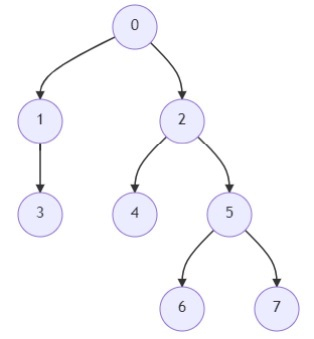# Program to find Kth ancestor of a tree node in Python

Suppose we have a tree with n nodes that are numbered from 0 to n-1. The tree is given by a parent array, where parent[i] is the parent of node i. The root of the tree is node 0. We have to find the kth ancestor of a given node, if the ancestor is not present, then return -1

So, if the input is likethen the output will be 2 because the first ancestor of node 6 is 5 and the second is 2.

To solve this, we will follow these steps −

• Define a function solve() . This will take parent, node, k

• if node is same as -1, then

• return -1

• otherwise when k is same as 1, then

• return parent[node]

• otherwise when (k AND k-1) is zero, then

• return solve(parent, solve(parent, node, quotient of k/2) , quotient of k/2)

• otherwise,

• msb := 2^(number of bits of k -1)

• return solve(parent, solve(parent, node, k-msb) , msb)

## Example

Let us see the following implementation to get better understanding

def solve(parent, node, k):
if node == -1:
return -1
elif k == 1:
return parent[node]
elif not (k & k-1):
return solve(parent, solve(parent, node, k >> 1), k >> 1)
else:
msb = 1 << (k.bit_length()-1)
return solve(parent, solve(parent, node, k-msb), msb)

parent = [-1,0,0,1,2,2,5,5]
node = 6
k = 2
print(solve(parent, node, k))

## Input

[6,7,9,16,22], 2

## Output

2